Example Questions

1 2 109 110 111 112 113 114 115 117 Next →

Example Question #12 : How To Solve For A Variable As Part Of A Fraction

Solve for x: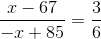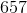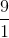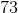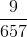Explanation:

In order to solve for x, you must cross multiply the ratio first. They will be equal to each other.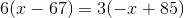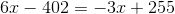, you then can add 3x to both sides and 402 to both sides as well. You are left with: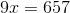divide both sides by 9 to get the final answer: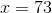Example Question #281 : Exponents

If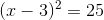, which of the following could be the value of?Explanation:Take the square root of both sides.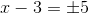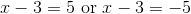Add 3 to both sides of each equation.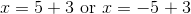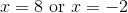Example Question #282 : Exponents

Simplify: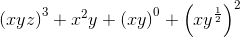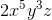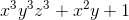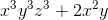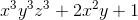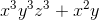Explanation:= x3y3z3 + x2y + x0y0 + x2y

x3y3z3 + x2y + 1 + x2y

x3y3z3 + 2x2y + 1

Example Question #6 : How To Use Foil With Exponents

Use the FOIL method to simplify the following expression: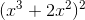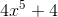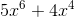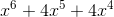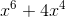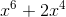Explanation:

Use the FOIL method to simplify the following expression:Step 1: Expand the expression.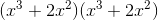Step 2: FOIL

First: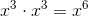Outside: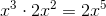Inside: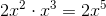Last: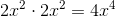Step 2: Sum the products.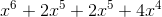Example Question #9 : How To Use Foil With Exponents

Square the binomial.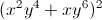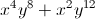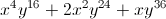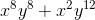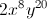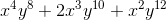Explanation: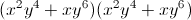We will need to FOIL.

First: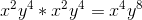Inside: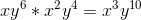Outside: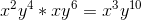Last: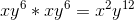Sum all of the terms and simplify.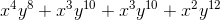Example Question #4 : How To Use Foil With Exponents

Which of the following is equivalent to 4c(3d)– 8c3d + 2(cd)4?

2cd(54d2 – 4c+ c* d3)

cd(54c * d– 4c+ c* d2)

2(54d– 4c+ 2c* d3)